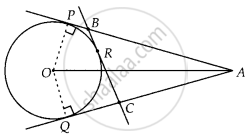# A is a point at a distance 13 cm from the centre O of a circle of radius 5 cm. AP and AQ are the tangents to the circle at P and Q. If a tangent BC is drawn at a point R lying on the minor arc PQ to - Mathematics

Sum

A is a point at a distance 13 cm from the centre O of a circle of radius 5 cm. AP and AQ are the tangents to the circle at P and Q. If a tangent BC is drawn at a point R lying on the minor arc PQ to intersect AP at B and AQ at C, find the perimeter of the ∆ABC.

#### SolutionOP ⊥ AP

∴ ∠OPA = 90°  ........[Tangent at any point of a circle is perpendicular to the radius through the point of contact]

In ∆OAP,

OA2 = OP2 + PA2

⇒ 132 = 52 + PA2

⇒ PA = 12 cm

Now, perimeter of ∆ABC = AB + BC + CA

= AB + BR + RC + CA

= (AB + BR) + (RC + CA)

= (AB + BP) + (CQ + CA)  .......[∵ BR = BP, RC = CQ i.e., tangents from external point to a circle are equal]

= AP + AQ

= 2AP  .......[∵ AP = AQ]

= 2 x 12

= 24 cm

Hence, the perimeter of ∆ABC = 24 cm.

Concept: Concept of Circle - Centre, Radius, Diameter, Arc, Sector, Chord, Segment, Semicircle, Circumference, Interior and Exterior, Concentric Circles
Is there an error in this question or solution?

#### APPEARS IN

NCERT Mathematics Exemplar Class 10
Chapter 9 Circles
Exercise 9.4 | Q 14 | Page 112
Share